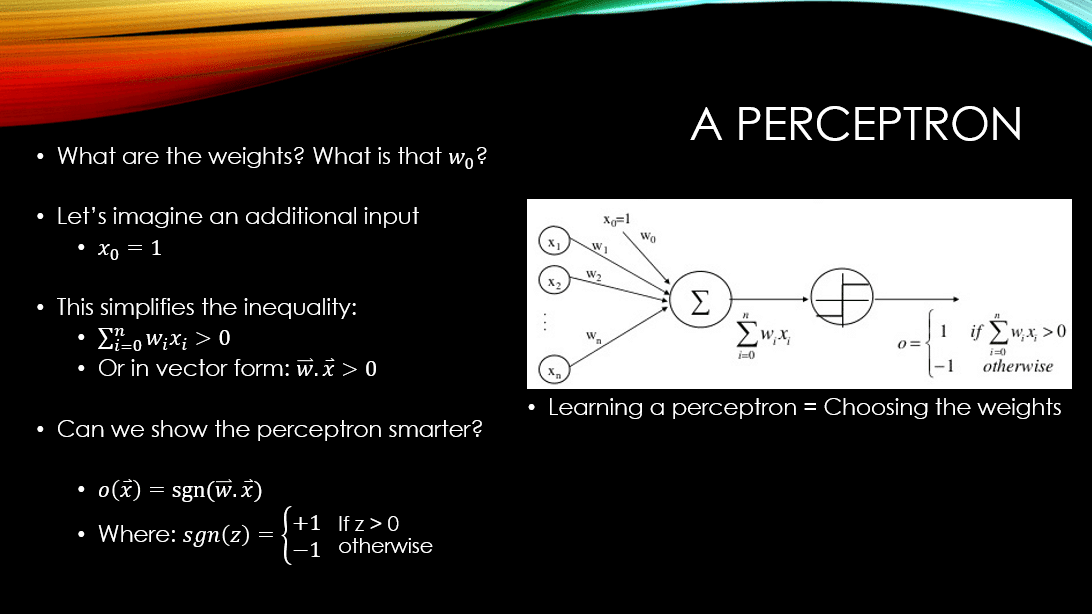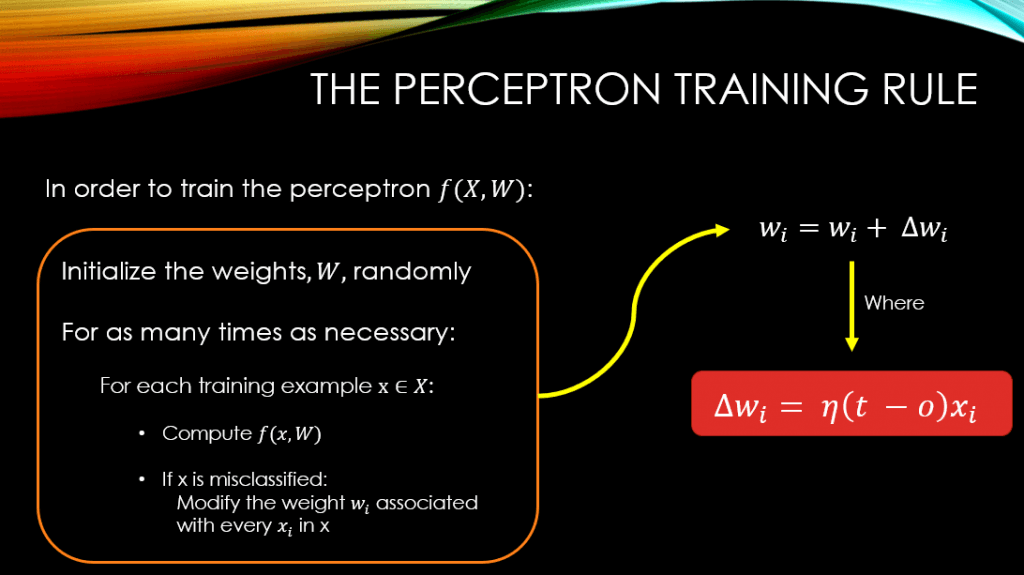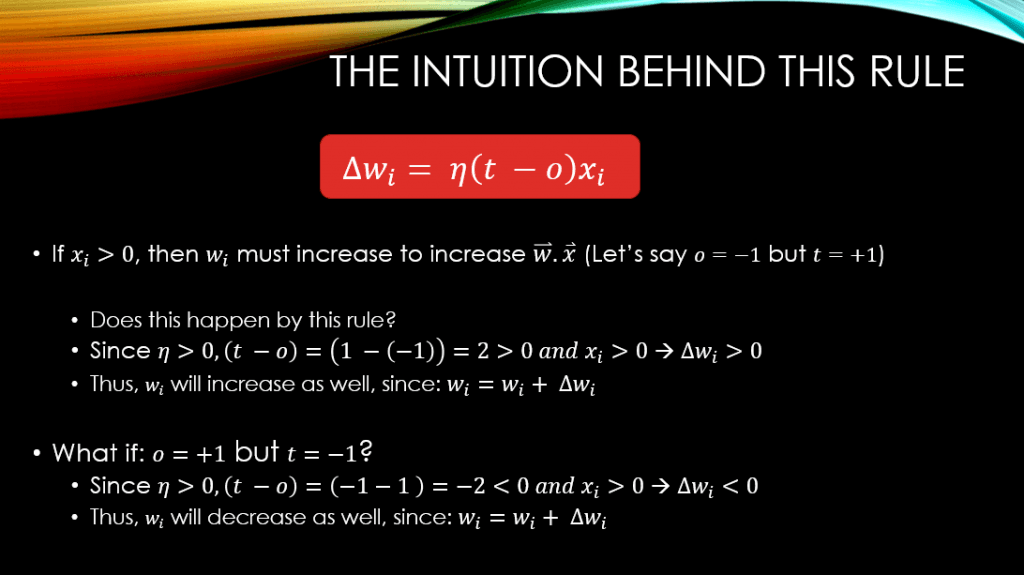## The Perceptron Training Rule

It is important to learn the training process of huge neural
networks. However, we need to simplify this by first understanding how a simple perceptron is trained, and how its weights are updated! Only then, will we be able to understand the dynamics of complicated and monstrous neural networks, such as CNNs, RNNs, MLPs, AENs, … .

In this post, you will learn one of the most fundamental
training techniques for training a simple perceptron and I will give you a nice intuition behind the math, so that you will learn this intuitively 😉

## The Case of Binary Classification

Below you can see the architecture of an actual perceptron, as the simplest type of artificial neural networks:Imagine we have a binary classification problem at hand, and we want to use a perceptron to learn this task. Moreover, as I have explained in my YouTube video, a perceptron can produce 2 values: +1 / -1 where +1 means that the input example belongs to the + class, and -1 means the input example belongs to the – class.

Obviously, as we have 2 classes, we would want to learn the weight vector of our perceptron in such a way that, for every training example (depending on whether it belongs to the + / – class), the perceptron would produce the correct +1 / -1. So, in summary:

What are we learning? The weights of the perceptron

Is any weight acceptable? Absolutely not! We would want to find a weight vector that makes the perceptron produce +1 for the + class, and -1 for the negative class

NOTE: You define which class is + and which is -! Moreover, you can train the perceptron and find a weight vector that produced +1 for – class and -1 for + class! It doesn’t really matter, as long as the perceptron can generate 2 different outputs for the instances that belong to class + / -. This is how you can measure the separating, and classification power of the perceptron.

So, we are talking supervised learning here, which means that we know the true class labels for every training example in our training set. As a result, in the perceptron training rule, we would initialize the weights at random and then feed the training examples into our perceptron and look at the produced outputthat can be either +1 or -1! So, we would want the perceptron to produce +1 for one class and -1 for the other. After observing the output for a given training example, we will NOT modify the weights unless the produced output was wrong! For example, if we want to produce +1 for + class and -1 for the – class, and if we fed an instance of the – class and the perceptron returned +1, then it means that we need to modify the parameters of our network, i.e., the weights. We will keep this process, and we will keep iterating through the training set as long as necessary until the perceptron classifies all the training examples correctly.

How do we update the weights? Is there some sort of mathematical rule? Yes, indeed there is! One such rule is called the perceptron training rule!

At every step of feeding a training example, when the perceptron fails to produce the correct +1/-1, we revise every weight wi associated with every input xi, according to the following rule:

wi = wi + Δwi

where:

Δwi = η(t – o)xi

• ####: This is thedimension of our input training example, which is connected to the weightThe training strategy of the perceptron is nicely demonstrated here:## The Intuition Behind the Perceptron Training Rule

There is a very nice intuition behind the perceptron training rule. If you are aking yourself, why does this rule have to learn a proper set of weight values for our perceptron, I applaud your question! I am going to give you an intuition behind the answer. Suppose our perceptron correctly classified a training example! Then clearly, we know that we will not need to change the weights of our perceptron! But does our learning rule confirm this as well? If the example has been classified correctly, then it means that (t – o) is 0! Why? Because when an example is classified correctly, the output of our perceptron is for sure equal to our ground truth, i.e., o = t!

Now let’s say the correct class was indeed the positive class where t =1, but our perceptron predicted the negative class, that is the output is -1, o = -1. So, looking at the figure of our perceptron, and knowing that for this particular example our perceptron has made a mistake, we realize that we need to change the weights in such a way that the output o would get closer to t. This means that we need to increase the value of the output, o. So, it seems that we need to increase the weights in such a way that w.x would increase! This way, if our input data are all positive xi > 0, then for sure increasing wi will bring the perceptron closer to correctly classifying this particular training example! Now, would you say our training rule would also follow our logic? Meaning, would it increase the wi? Well, in this case (t – o), η, and xi are all positive, so Δwi is also positive, which means that we are increasing the old value of wi positively.I hope that this has been informative for you,

On behalf of MLDawn,

Take care of yourselves 😉